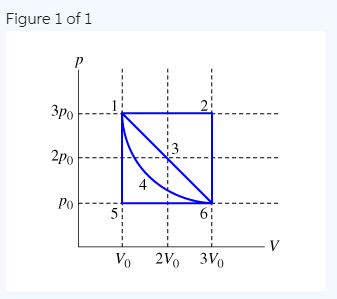# Problem: Calculate the work W done by the gas during process 1→2→6→5→1.One important use for pV diagrams is in calculating work. The product pV has the units of Pa×m3=(N/m2)⋅m3=N⋅m=J; in fact, the absolute value of the work done by the gas (or on the gas) during any process equals the area under the graph corresponding to that process on the pV diagram. If the gas increases in volume, it does positive work; if the volume decreases, the gas does negative work (or, in other words, work is being done on the gas). If the volume does not change, the work done is zero.

###### FREE Expert Solution

Work done:

$\overline{){\mathbf{W}}{\mathbf{=}}{\mathbf{P}}{\mathbf{·}}{\mathbf{∆}}{\mathbf{V}}}$

P12 = 3P0

ΔV12 = Vf - V0 = V2 - V1 = 3V0 - V0 = 2V0

W12 = P12•ΔV12 = 3P0•2V0 = 6P0V0

ΔV26 = Vf - V0 = V6 - V2 = 3V0 - 3V0 = 0

W26 = P26•ΔV26 = 3P0•0 = 0

86% (15 ratings)###### Problem Details

Calculate the work W done by the gas during process 1→2→6→5→1.

One important use for pV diagrams is in calculating work. The product pV has the units of Pa×m3=(N/m2)⋅m3=N⋅m=J; in fact, the absolute value of the work done by the gas (or on the gas) during any process equals the area under the graph corresponding to that process on the pV diagram. If the gas increases in volume, it does positive work; if the volume decreases, the gas does negative work (or, in other words, work is being done on the gas). If the volume does not change, the work done is zero.